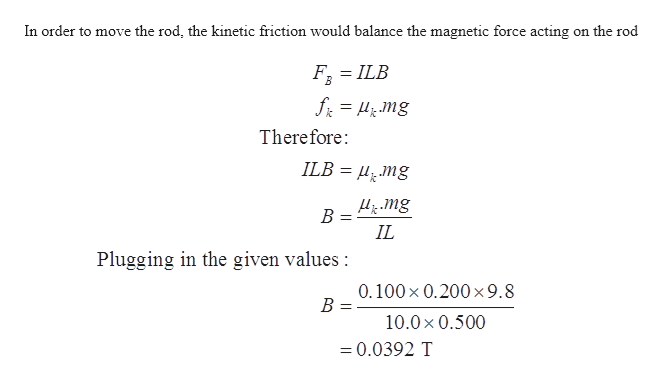# A 0.200 - kg metal rod carrying a current of 10.0 A glides on twohorizontal rails 0.500 m apart. What vertical magnetic field isrequired to keep the rod moving at a constant speed if the coefficientof kinetic friction between the rod and rails is 0.100?

Question
4 views

A 0.200 - kg metal rod carrying a current of 10.0 A glides on two
horizontal rails 0.500 m apart. What vertical magnetic field is
required to keep the rod moving at a constant speed if the coefficient
of kinetic friction between the rod and rails is 0.100?

check_circle

Step 1

Given data:

Mass of the metal rod, m =0.200 kg

Current in the rod, I = 10.0 A

Separation between the rod, L = 0.500 m

Coefficient of kinetic friction = 0.100

Step 2

In order to move the rod, the kinetic friction would ...help_outlineImage TranscriptioncloseIn order to move the rod, the kinetic friction would balance the magnetic force acting on the rod = ILB f = H; mg Therefore: ILB = µ̟Mg Hi mg B = IL %3D Plugging in the given values : 0.100 x 0.200x9.8 10.0x 0.500 = 0.0392 T fullscreen

### Want to see the full answer?

See Solution

#### Want to see this answer and more?

Solutions are written by subject experts who are available 24/7. Questions are typically answered within 1 hour.*

See Solution
*Response times may vary by subject and question.
Tagged in
SciencePhysics

### Other